# SSAT Middle Level Math : How to multiply fractions

## Example Questions

### Example Question #11 : Concepts

Evaluate: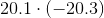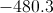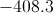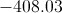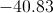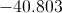Explanation:

The product of two numbers of unlike sign is the (negative) opposite of the product of their absolute values.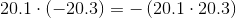There are a total of two digits to the right of the two decimal points. Therefore, the product can be calculated by multiplying 201 by 203, then placing the decimal point so that there are three digits at right.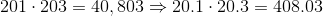Since we need to affix a negative symbol in front, the answer is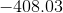### Example Question #21 : How To Multiply Fractions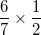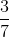Explanation:

When we multiply fractions, we multiply the numerator by the numerator and the denominator by the denominator.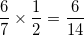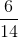can be reduced by dividing both sides by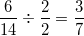### Example Question #21 : How To Multiply Fractions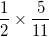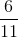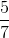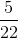Explanation:

When we multiply fractions, we multiply the numerator by the numerator and the denominator by the denominator.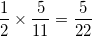### Example Question #1 : Multiply A Fraction Or Whole Number By A Fraction: Ccss.Math.Content.5.Nf.B.4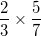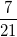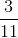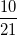Explanation:

When we multiply fractions, we multiply the numerator by the numerator and the denominator by the denominator.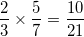### Example Question #22 : How To Multiply Fractions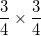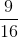Explanation:

When we multiply fractions, we multiply the numerator by the numerator and the denominator by the denominator.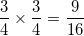### Example Question #261 : Fractions

Solve: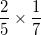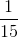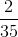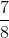Explanation:

When we multiply fractions, we multiply the numerator by the numerator and the denominator by the denominator.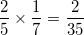### Example Question #11 : Multiply A Fraction Or Whole Number By A Fraction: Ccss.Math.Content.5.Nf.B.4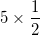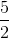Explanation:When we multiply a fraction by a whole number, we first want to make the whole number into a fraction. We do that by putting the whole number over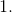The we multiply like normal.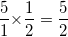### Example Question #12 : Multiply A Fraction Or Whole Number By A Fraction: Ccss.Math.Content.5.Nf.B.4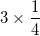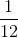Explanation:When we multiply a fraction by a whole number, we first want to make the whole number into a fraction. We do that by putting the whole number overThen we multiply like normal.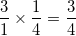### Example Question #21 : How To Multiply Fractions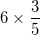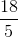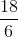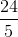Explanation:When we multiply a fraction by a whole number, we first want to make the whole number into a fraction. We do that by putting the whole number overThen we multiply like normal.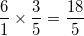### Example Question #21 : Multiply A Fraction Or Whole Number By A Fraction: Ccss.Math.Content.5.Nf.B.4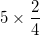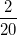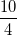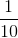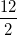Explanation:When we multiply a fraction by a whole number, we first want to make the whole number into a fraction. We do that by putting the whole number overThen we multiply like normal.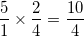### All SSAT Middle Level Math Resources